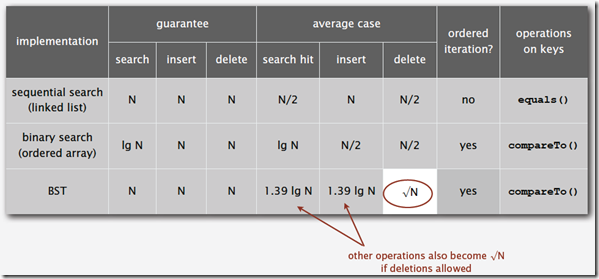# 一 定义

1. 若任意节点的左子树不空，则左子树上所有结点的值均小于它的根结点的值；

2. 若任意节点的右子树不空，则右子树上所有结点的值均大于它的根结点的值；

3. 任意节点的左、右子树也分别为二叉查找树。

4. 没有键值相等的节点（no duplicate nodes）。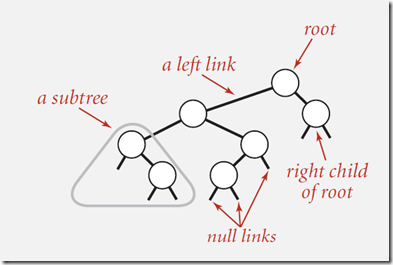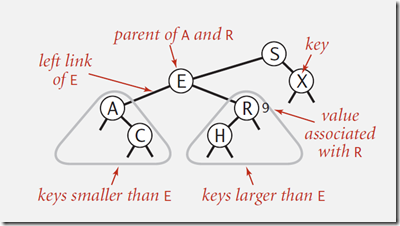# 二 实现

``````public class BinarySearchTreeSymbolTable<TKey, TValue> : SymbolTables<TKey, TValue> where TKey : IComparable<TKey>, IEquatable<TValue>
{
private Node root;
private class Node
{
public Node Left { get; set; }
public Node Right { get; set; }
public int Number { get; set; }
public TKey Key { get; set; }
public TValue Value { get; set; }

public Node(TKey key, TValue value, int number)
{
this.Key = key;
this.Value = value;
this.Number = number;
}
}
...
}
``````

## 查找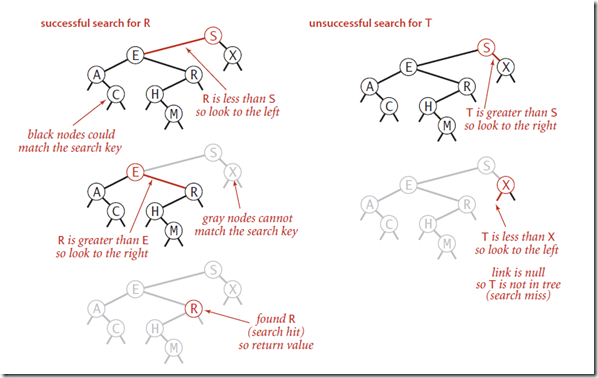``````public override TValue Get(TKey key)
{
TValue result = default(TValue);
Node node = root;
while (node != null)
{

if (key.CompareTo(node.Key) > 0)
{
node = node.Right;
}
else if (key.CompareTo(node.Key) < 0)
{
node = node.Left;
}
else
{
result = node.Value;
break;
}
}
return result;
}
``````

``````public TValue Get(TKey key)
{
return GetValue(root, key);
}

private TValue GetValue(Node root, TKey key)
{
if (root == null) return default(TValue);
int cmp = key.CompareTo(root.Key);
if (cmp > 0) return GetValue(root.Right, key);
else if (cmp < 0) return GetValue(root.Left, key);
else return root.Value;
}
``````

## 插入

``````public override void Put(TKey key, TValue value)
{
root = Put(root, key, value);
}

private Node Put(Node x, TKey key, TValue value)
{
//如果节点为空，则创建新的节点，并返回
//否则比较根据大小判断是左节点还是右节点，然后继续查找左子树还是右子树
//同时更新节点的Number的值
if (x == null) return new Node(key, value, 1);
int cmp = key.CompareTo(x.Key);
if (cmp < 0) x.Left = Put(x.Left, key, value);
else if (cmp > 0) x.Right = Put(x.Right, key, value);
else x.Value = value;
x.Number = Size(x.Left) + Size(x.Right) + 1;
return x;
}

private int Size(Node node)
{
if (node == null) return 0;
else return node.Number;
}
``````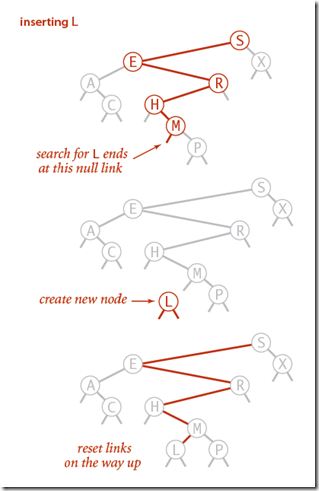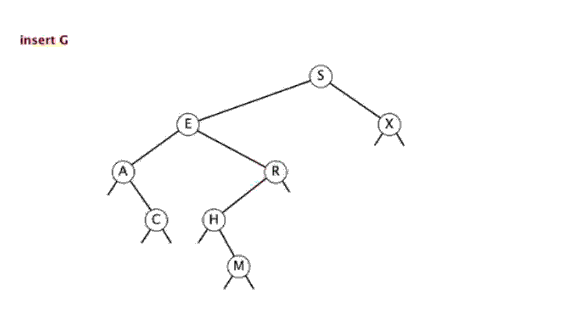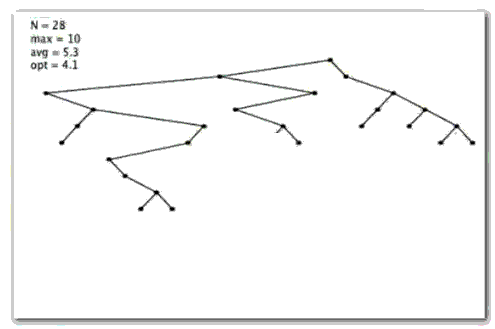## 最大最小值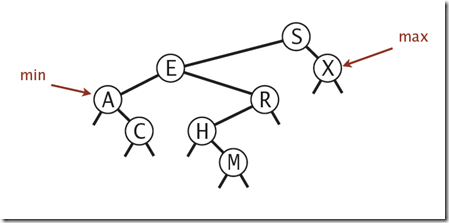``````public override TKey GetMax()
{
TKey maxItem = default(TKey);
Node s = root;
while (s.Right != null)
{
s = s.Right;
}
maxItem = s.Key;
return maxItem;
}

public override TKey GetMin()
{
TKey minItem = default(TKey);
Node s = root;
while (s.Left != null)
{
s = s.Left;
}
minItem = s.Key;
return minItem;
}
``````

``````public TKey GetMaxRecursive()
{
return GetMaxRecursive(root);
}

private TKey GetMaxRecursive(Node root)
{
if (root.Right == null) return root.Key;
return GetMaxRecursive(root.Right);
}

public TKey GetMinRecursive()
{
return GetMinRecursive(root);
}

private TKey GetMinRecursive(Node root)
{
if (root.Left == null) return root.Key;
return GetMinRecursive(root.Left);
}
``````

## Floor和Ceiling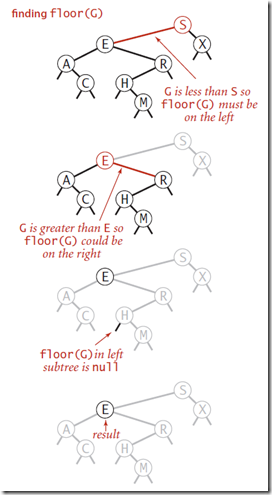``````public TKey Floor(TKey key)
{
Node x = Floor(root, key);
if (x != null) return x.Key;
else return default(TKey);
}

private Node Floor(Node x, TKey key)
{
if (x == null) return null;
int cmp = key.CompareTo(x.Key);
if (cmp == 0) return x;
if (cmp < 0) return Floor(x.Left, key);
else
{
Node right = Floor(x.Right, key);
if (right == null) return x;
else return right;
}
}
``````

## 删除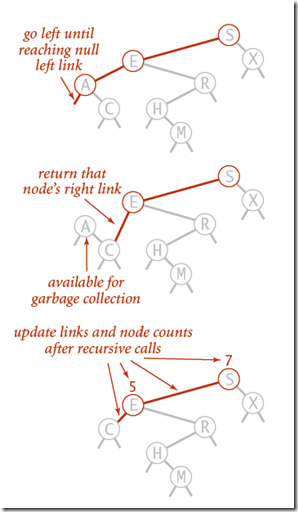``````public void DelMin()
{
root = DelMin(root);
}

private Node DelMin(Node root)
{
if (root.Left == null) return root.Right;
root.Left = DelMin(root.Left);
root.Number = Size(root.Left) + Size(root.Right) + 1;
return root;
}
``````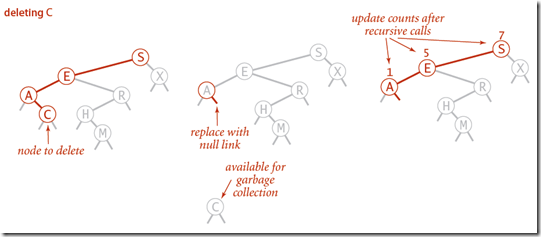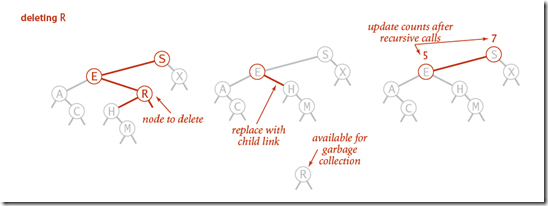\1. 保存带删除的节点到临时变量t

\2. 将t的右节点的最小节点min(t.right)保存到临时节点x

\3. 将x的右节点设置为deleteMin(t.right)，该右节点是删除后，所有比x.key最大的节点。

\4. 将x的做节点设置为t的左节点。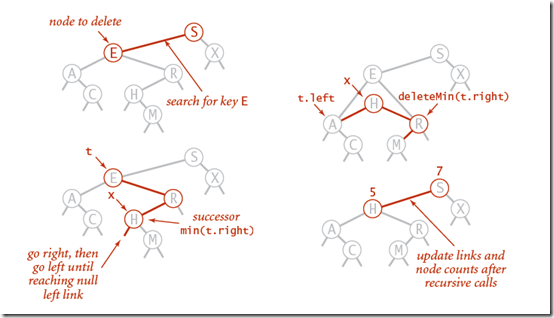``````public void Delete(TKey key)
{
root =Delete(root, key);

}

private Node Delete(Node x, TKey key)
{
int cmp = key.CompareTo(x.Key);
if (cmp > 0) x.Right = Delete(x.Right, key);
else if (cmp < 0) x.Left = Delete(x.Left, key);
else
{
if (x.Left == null) return x.Right;
else if (x.Right == null) return x.Left;
else
{
Node t = x;
x = GetMinNode(t.Right);
x.Right = DelMin(t.Right);
x.Left = t.Left;
}
}
x.Number = Size(x.Left) + Size(x.Right) + 1;
return x;
}

private Node GetMinNode(Node x)
{
if (x.Left == null) return x;
else return GetMinNode(x.Left);
}
``````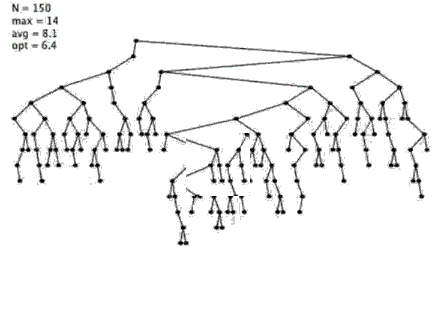# 三 分析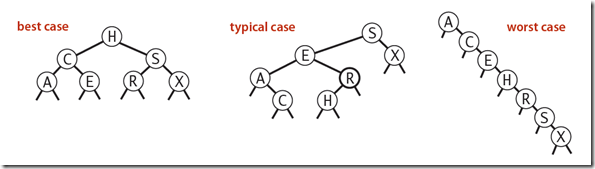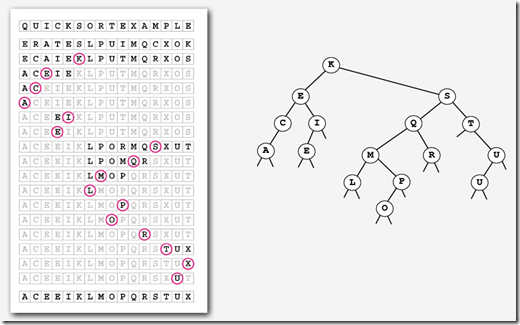BST中位于顶部的元素就是快速排序中的第一个划分的元素，该元素左边的元素全部小于该元素，右边的元素均大于该元素。

# 四 遍历方式

• 二叉树的遍历（traversing binary tree）是指从根结点出发，按照某种次序依次访问二叉树中所有的结点，使得每个结点被访问依次且仅被访问一次。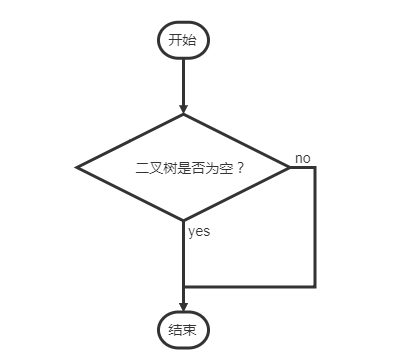• 前序遍历

• 中序遍历

• 后续遍历

• 层序遍历

# 五 总结